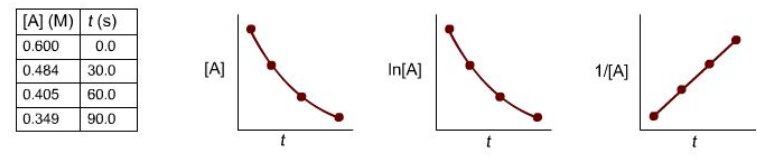Chemistry Practice Problems Identifying Reaction Order Practice Problems Solution: For A → products, time and concentration data were...

🤓 Based on our data, we think this question is relevant for Professor Feakes' class at TXSTATE.

# Solution: For A → products, time and concentration data were collected and plotted as shown below.Determine the reaction order, the rate constant, and the units of the rate constant.

###### Problem

For A → products, time and concentration data were collected and plotted as shown below.

Determine the reaction order, the rate constant, and the units of the rate constant.Identifying Reaction Order

Identifying Reaction Order

#### Q. The reaction was monitored a function of time:A → B + CA plot of ln[A] versus time yields a straight line with slope -0.0045/s.a. What is the value of...

Solved • Thu Mar 15 2018 10:48:39 GMT-0400 (EDT)

Identifying Reaction Order

#### Q. The following reaction was monitored as a function of time:AB → A + BA plot of 1/[AB] versus time yields a straight line with slope 5.5 × 10 −2 (M⋅s)−...

Solved • Tue Mar 13 2018 13:11:51 GMT-0400 (EDT)

Identifying Reaction Order

#### Q. Identify the order with respect to the reactant, A.  (A → product) i) The half-life of A increases as the initial concentration of A decreases.   ...

Solved • Mon Feb 12 2018 16:43:01 GMT-0500 (EST)

Identifying Reaction Order

#### Q. For the reaction:X → Y Identify what the graphs of [X] versus time and [Y] versus time would look like for various orders.A) Zero-order [X] vs. tB) Ze...

Solved • Fri Dec 22 2017 14:24:40 GMT-0500 (EST)